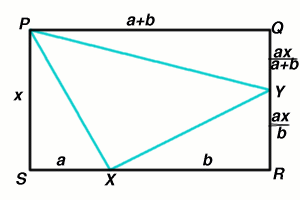#### You may also like### Darts and Kites

Explore the geometry of these dart and kite shapes!### Pent

The diagram shows a regular pentagon with sides of unit length. Find all the angles in the diagram. Prove that the quadrilateral shown in red is a rhombus.### Pentakite

ABCDE is a regular pentagon of side length one unit. BC produced meets ED produced at F. Show that triangle CDF is congruent to triangle EDB. Find the length of BE.

# Golden Thoughts

##### Age 14 to 16Challenge Level

Sue from Madras College, St Andrew's, Scotland sent in a good solution to this problem.Let $SX = a$ and $XR= b$ then $PQ=a+b$ and, since the areas of the triangles $SPX, XYR$ and $PQY$ are equal, we know that $YR= ax/b$ and $QY = ax/(a+b)$. Because $PS = QR$ we now have $$\frac{ax}{a+b} + \frac{ax}{b} = x$$ and simplifying this we get $b^2 -ab - a^2 = 0$. So, writing $t$ for $\frac{b}{a}$, we get the quadratic equation $$t^2 - t - 1 = 0$$ which has roots $$t = \frac{1 \pm \sqrt 5}{2}$$ but we know that $t$ is positive so $$t =\frac {1 + \sqrt 5}{2}.$$ Also, writing the ratio $RY/YQ$ in terms of $t$ we get $$\frac{RY}{YQ} = \frac{a+b}{b} = \frac {1}{t} + 1.$$ We already know that $t=\frac{1}{t} + 1$ because $t^2 = 1 + t$. So $$\frac{RX}{XS} = \frac {RY}{YQ} = \frac {1 + \sqrt 5}{2}.$$